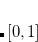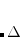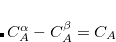Q-Chem 5.1 User’s Manual

# C.1 Q-Chem Text Input Summary

• Users are able to enter input sections in any order; see Table 3.1 for a complete list of input sections.

• Each input section must be terminated with $end. • Not all input sections are required, but$rem and $molecule are compulsory. • The entire Q-Chem input is case-insensitive. • Multiple jobs are separated by the string @@@ on a single line. ## C.1.1 Keyword:$molecule

Four methods are available for inputing geometry information:

• Z-matrix (Ångstroms and degrees):
$molecule {Z-matrix} {blank line, if parameters are being used} {Z-matrix parameters, if used}$end

• Cartesian Coordinates (Ångstroms):
$molecule {Cartesian coordinates} {blank line, if parameter are being used} {Coordinate parameters, if used}$end

• Read from a previous calculation:
$molecule read$end

$molecule read filename$end

## C.1.2 Keyword: $rem See also the list of$rem variables at the end of this Appendix. The general format is:

$rem REM_VARIABLE VALUE [optional comment]$end


## C.1.4 Keyword: $comment Note that the entire input deck is echoed to the output file, thus making the$comment keyword largely redundant.

$comment User comments - copied to output file$end


## C.1.5 Keyword: $ecp$ecp
For each atom that will bear an ECP
Chemical symbol for the atom
ECP name; thevalue for the ECP; number of core electrons removed
For each ECP component (in the order unprojected,,, ,The component name
The number of Gaussians in the component
For each Gaussian in the component
The power of; the exponent; the contraction coefficient
****
$end Note: (1) All of the information in the$ecp block is case–insensitive.
(2) Thevalue may not exceed 4. That is, nothing beyondprojectors is allowed.
(3) The power of(which includes the Jacobianfactor) must be 0, 1 or 2.

## C.1.6 Keyword: $empirical_dispersion $empirical_dispersion
S6 S6_value
D D_value
C6 element_1 C6_value_for_element_1 element_2 C6_value_for_element_2
$end  Note: This section is only for values that the user wants to change from the default values recommended by Grimme. ## C.1.7 Keyword:$external_charges

All input should be given in atomic units.

Update: While charges should indeed be listed in atomic units, the units for distances depend on the user input. If the structure is specified in Ångstroms (the default), the coordinates for external charges should also be in Ångstroms. If the structure is specified in atomic units, the coordinates for external charges should also be in atomic units. (See INPUT_BOHR.)

$external_charges x-coord1 y-coord1 z-coord1 charge1 x-coord2 y-coord2 z-coord2 charge2$end


## C.1.8 Keyword: $intracule$intracule
 int_type 0 Computeonly 1 Computeonly 2 Computeonly 3 Compute,and4 Computeand5 Computeand6 Computeandu_points Number of points, start, end. v_points Number of points, start, end. moments 0–4 Order of moments to be computed (only). derivs 0–4 order of derivatives to be computed (only). accuracy(specify accuracy of intracule interpolation table (only).
$end ## C.1.9 Keyword:$isotopes

Note that masses should be given in atomic units.

$isotopes number_extra_loops tp_flag number_of_atoms [temp pressure] atom_number1 mass1 atom_number2 mass2 ...$end


## C.1.10 Keyword: $multipole_field Multipole fields are all in atomic units. $multipole_field
field_component1   value1
field_component2   value2
...
$end  ## C.1.11 Keyword:$nbo

Refer to Chapter 11 and the NBO manual for further information. Note that the NBO $rem variable must be set to ON to initiate the NBO package. $nbo
[ NBO options ]
$end  ## C.1.12 Keyword:$occupied

$occupied 1 2 3 4 ... nalpha 1 2 3 4 ... nbeta$end


## C.1.13 Keyword: $opt Note that units are in Ångstroms and degrees. Also see the summary in the next section of this Appendix. $opt
CONSTRAINT
stre  atom1  atom2  value
...
bend  atom1  atom2  atom3  value
...
outp  atom1  atom2  atom3  atom4  value
...
tors  atom1  atom2  atom3  atom4  value
...
linc  atom1  atom2  atom3  atom4  value
...
linp  atom1  atom2  atom3  atom4  value
...
ENDCONSTRAINT

FIXED
atom   coordinate_reference
...
ENDFIXED

DUMMY
idum   type   list_length   defining_list
...
ENDDUMMY

CONNECT
atom   list_length   list
...
ENDCONNECT
$end  ## C.1.14 Keyword:$svp

$svp <KEYWORD>=<VALUE>, <KEYWORD>=<VALUE>,... <KEYWORD>=<VALUE>$end


For example, the section may look like this:

$svp RHOISO=0.001, DIELST=78.39, NPTLEB=110$end


## C.1.15 Keyword: $svpirf $svpirf
<# point> <x point> <y point> <z point> <charge> <grid weight>
<# point> <x normal> <y normal> <z normal>
$end  ## C.1.16 Keyword:$plots

$plots One comment line Specification of the 3–D mesh of points on 3 lines:A line with 4 integers indicating how many things to plot:An optional line with the integer list of MO’s to evaluate (only if) MO(1) MO(2)MO() An optional line with the integer list of densities to evaluate (only if) Rho(1) Rho(2)Rho() An optional line with the integer list of transition densities (only if) Trans(1) Trans(2)Trans() An optional line with states for detachment/attachment densities (if) DA(1) DA(2)DA()$end

## C.1.17 Keyword: $localized_diabatization$plots
One comment line.
One line with an an array of adiabatic states to mix together.$end Note: We count adiabatic states such that the first excited state is, the fifth is, and so forth. ## C.1.18 Keyword:$van_der_waals

Note: All radii are given in Ångstroms.

$van_der_waals 1 atomic_number VdW_radius$end


(alternative format)

$van_der_waals 2 sequential_atom_number VdW_radius$end


## C.1.19 Keyword: $xc_functional $xc_functional
X  exchange_symbol  coefficient
X  exchange_symbol  coefficient
...
C  correlation_symbol  coefficient
C  correlation_symbol  coefficient
...
K  coefficient
\$end